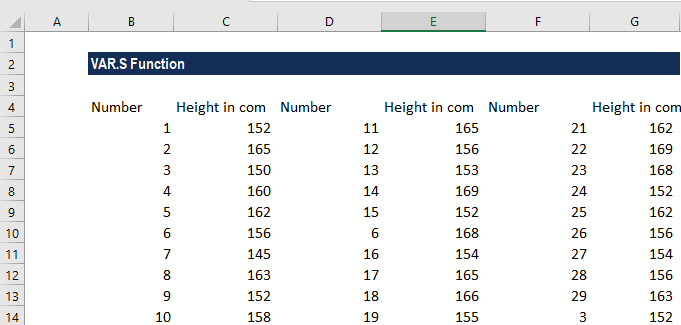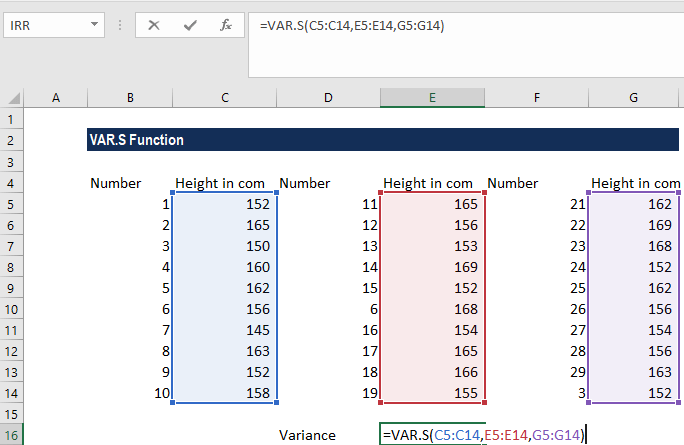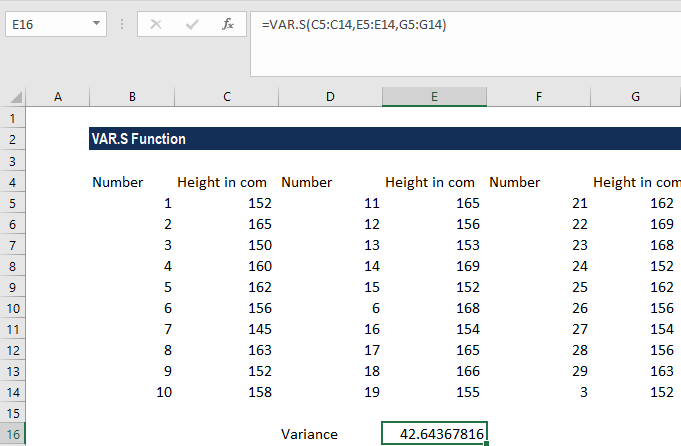# VAR.S Function

Returns the variance of a sample

## What is the VAR.S Function?

The VAR.S function is categorized under Excel Statistical functions. This function will return the variance of a sample. It was introduced in Excel’s 2010 version.

As we know, variance is a statistical measure used across a set of values to identify the amount that the values vary from the average value.

In financial analysis, variance is useful in determining the risk(s) undertaken by an investor. Most portfolio managers use it for determining the allocation of their clients’ assets/investments.

### Formula

=VAR.S(number1,[number2],…)

The VAR.S function uses the following arguments:

1. Number1 (required argument) – This is the first argument corresponding to a sample of a population.
2. Number 2,…  (optional argument) – Here, the number arguments can be up to 254 values or arrays of values that provide at least two values to the function.

When providing the arguments, we need to remember that:

1. Arguments can either be numbers or names, arrays, or references that contain numbers.
2. Logical values and text representations of numbers that are typed directly into the list of arguments are counted by this function.
3. If an argument is an array or reference, only numbers in that array or reference are counted. Empty cells, logical values, text, or error values in the array or reference are ignored.

### How to use VAR.S Function in Excel?

As a worksheet function, VAR.S can be entered as part of a formula in a cell of a worksheet. To understand the uses of the function, let’s consider an example:

#### Example

Suppose we are given data about the height of children from three cities, as shown below:For variance, the formula used is:We get the results below:In the above example, we took the arguments for the VAR.S function as input in the form of three cell ranges. However, we can also enter the figures directly, either as individual numbers or number arrays.

### A few things to remember about the VAR.S Function

1. #DIV/0 error – Occurs if we provided less than two numeric values.
2. #VALUE! error – Occurs when any of the values provided directly to this function are text values that cannot be interpreted as numbers.
3. VAR.S assumes the arguments are a sample of data, not an entire population. If the data represents an entire population, use VAR.P.
4. If we wish to evaluate logical values and/or text in references, we should use the VARA function.

Thanks for reading CFI’s guide to the Excel VAR.S function. By taking the time to learn and master these functions, you’ll significantly speed up your financial analysis and modeling. To learn more, check out these additional CFI resources:

• Excel for Finance